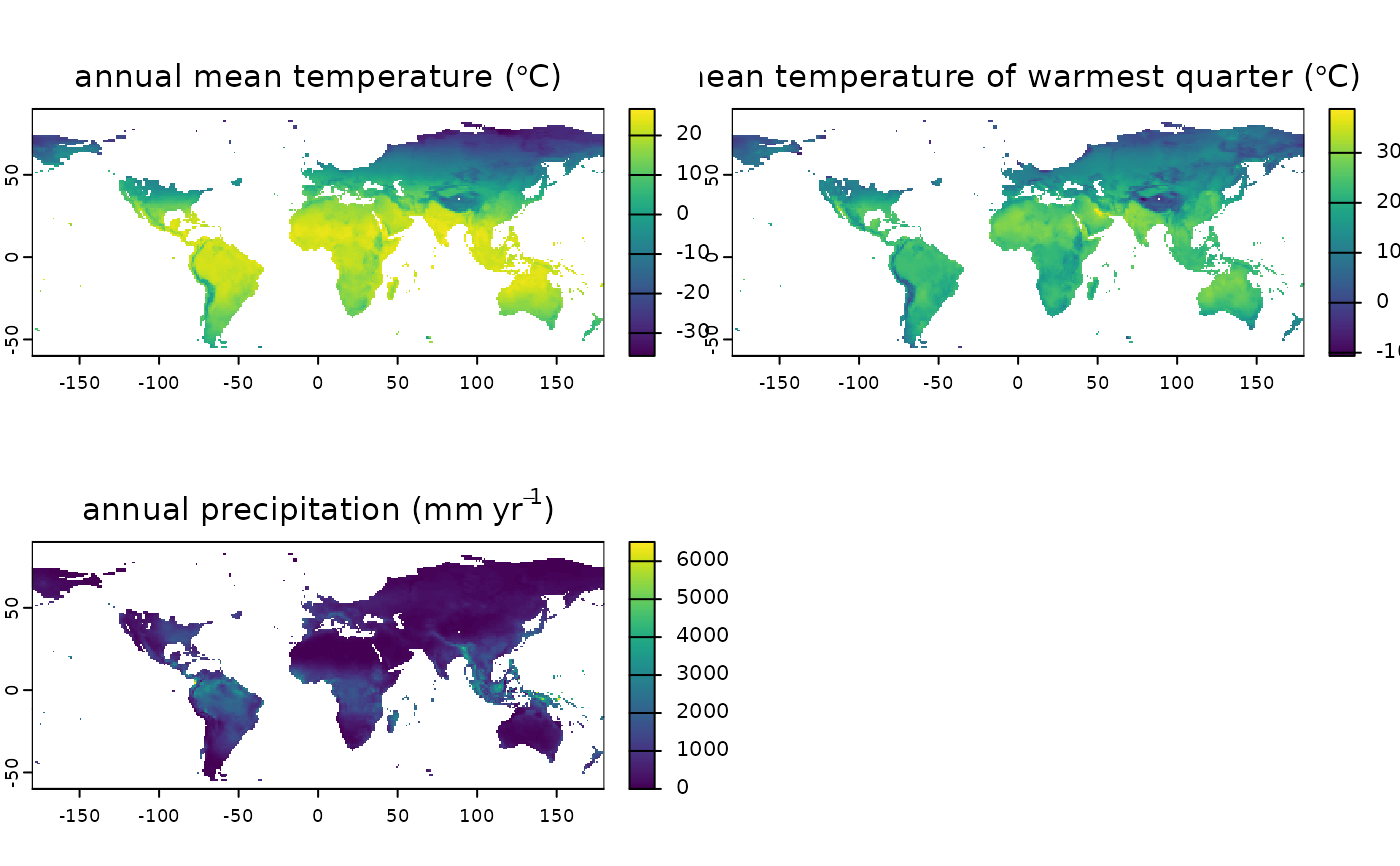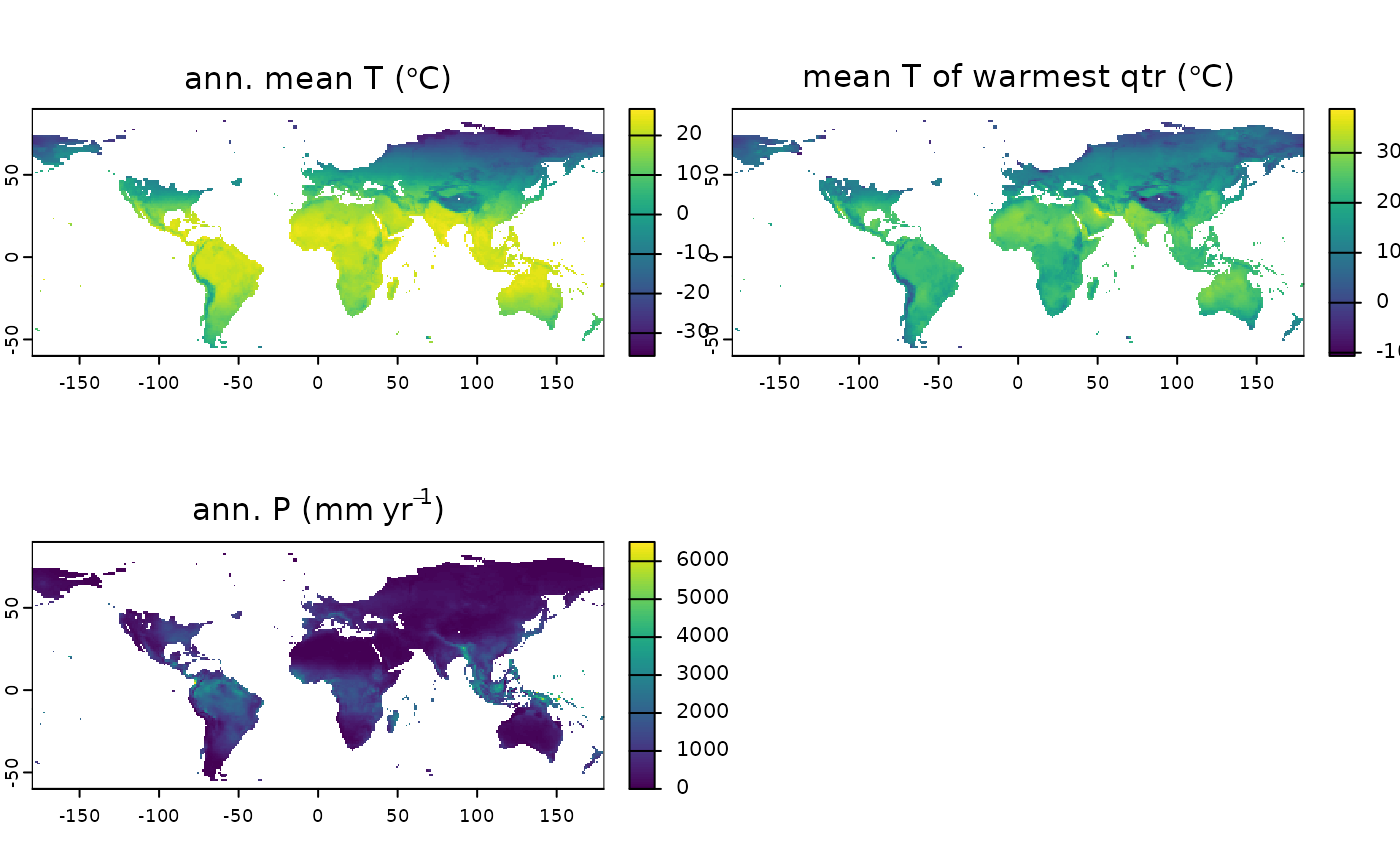Generate pretty labels (in the form of an expression) that can be used for plotting

## Usage

var_labels(x, dataset, with_units = TRUE, abbreviated = FALSE)

## Arguments

x

either a character vector with the names of the variables, or a terra::SpatRaster generated with [region_slice())]

[region_slice())]: R:region_slice())

dataset

string defining dataset to be downloaded (a list of possible values can be obtained with list_available_datasets()). This function will not work on custom datasets.

with_units

boolean defining whether the label should include units

abbreviated

boolean defining whether the label should use abbreviations for the variable

## Value

a expression that can be used as a label in plots

## Examples

var_labels("bio01", dataset = "Example")
#> expression("annual mean temperature (" * degree * C * ")")

# set the data_path for this example to run on CRAN
# users don't need to run this line
set_data_path(on_CRAN = TRUE)
#>  TRUE

# for a SpatRaster
climate_20k <- region_slice(
time_bp = -20000,
bio_variables = c("bio01", "bio10", "bio12"),
dataset = "Example"
)
terra::plot(climate_20k, main = var_labels(climate_20k, dataset = "Example"))terra::plot(climate_20k, main = var_labels(climate_20k,
dataset = "Example",
abbreviated = TRUE
))